Courses

# Point of Inflection - Differentiation, Business Mathematics & Statistics B Com Notes | EduRev

## B Com : Point of Inflection - Differentiation, Business Mathematics & Statistics B Com Notes | EduRev

The document Point of Inflection - Differentiation, Business Mathematics & Statistics B Com Notes | EduRev is a part of the B Com Course Business Mathematics and Statistics.
All you need of B Com at this link: B Com

## Inflection Points

An Inflection Point is where a curve changes from Concave upward to Concave downward (or vice versa)

So what is concave upward / downward ?
Concave upward is when the slope increases: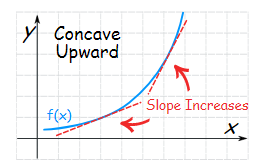Concave upward is when the slope decreases: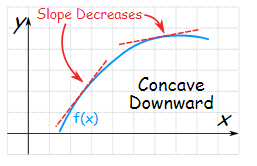Here are some more examples: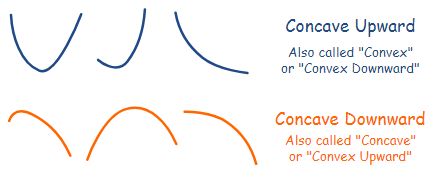## Finding where ...

So our task is to find where a curve goes from concave upward to concave downward (or vice versa).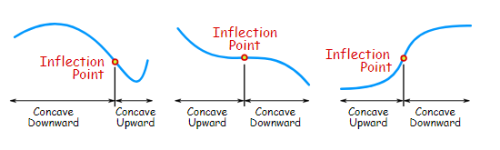## Calculus

Derivatives help us!

The derivative of a function gives the slope.

The second derivative tells us if the slope increases or decreases.

• When the second derivative is positive, the function is concave upward.
• When the second derivative is negative, the function is concave downward.

And the inflection point is where it goes from concave upward to concave downward (or vice versa).
Example: y = 5x3 + 2x2 − 3x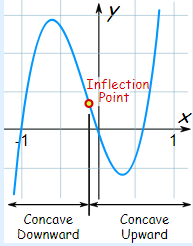Let's work out the second derivative:

• The derivative is y' = 15x2 + 4x − 3
• The second derivative is y'' = 30x + 4

And 30x + 4 is negative up to x = −4/30 = −2/15, positive from there onwards. So:

f(x) is concave downward up to x = −2/15

f(x) is concave upward from x = −2/15 on

And the inflection point is at x = −2/15

### A Quick Refresher on Derivatives

In the previous example we took this:

y = 5x3 + 2x2 − 3x

and came up with this derivative:

y' = 15x2 + 4x − 3

There are rules you can follow to find derivatives, and we used the "Power Rule":

• x3 has a slope of 3x2, so 5x3 has a slope of 5(3x2) = 15x2
• x2 has a slope of 2x, so 2x2 has a slope of 2(2x) = 4x
• The slope of the line 3x is 3

Another example for you:

### Example: y = x3 − 6x2 + 12x − 5

The derivative is: y' = 3x2 − 12x + 12

The second derivative is: y'' = 6x − 12

And 6x − 12 is negative up to x = 2, positive from there onwards. So:

f(x) is concave downward up to x = 2

f(x) is concave upward from x = 2 on

And the inflection point is at x = 2: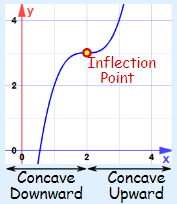122 videos|142 docs

,

,

,

,

,

,

,

,

,

,

,

,

,

,

,

,

,

,

,

,

,

,

,

,

;